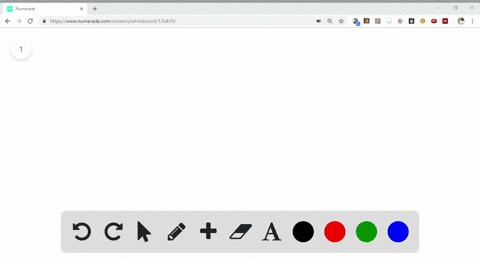Sign up for our free STEM online summer camps starting June 1st!View Summer Courses### What is the molecular formula of each of the foll…

06:22University of New Mexico

Need more help? Fill out this quick form to get professional live tutoring.

Get live tutoring
Problem 51

What is the molecular formula of each of the following compounds?
$$\begin{array}{l}{\text { (a) empirical formula } \mathrm{CH}_{2}, \text { molar mass }=84.0 \mathrm{g} / \mathrm{mol}} \\ {\text { (b) empirical formula } \mathrm{NH}_{2} \mathrm{Cl} \text { , molar mass }=51.5 \mathrm{g} / \mathrm{mol}}\end{array}$$

a) $C 6 H 12$
b) See explanation for solution.

## Discussion

You must be signed in to discuss.

## Video Transcript

Okay, so the sub script in the molecule formula off a substance are always the bowl number multiplies this off script in the empirical formula. Using this The empirical formula given his ch two on the molar mass is 84 grams. The empirical formula is this. Some off atomic beats off atoms in the compound. So the empirical formula V eight will be 14. That is 12 for carbon and one for hydrogen de Voll. Number multiple is six. So my deep link the empirical formula with six give us the molecular formula as C six extra for compound to be. We have the molecule. We have the empirical formula as image to seal on the molar mass as 51.5 grams. The mask off empirical formula alone is 51.5. Atomic wants unit on de Voll number multiple is also one hands. Nothing will be changed in this option. The empirical formula is seen as the molecule of formula